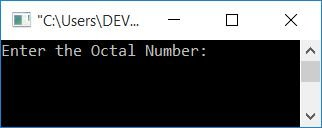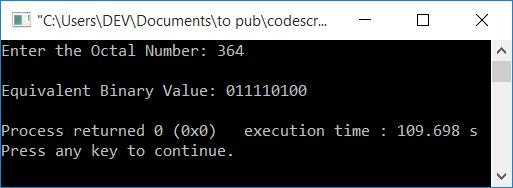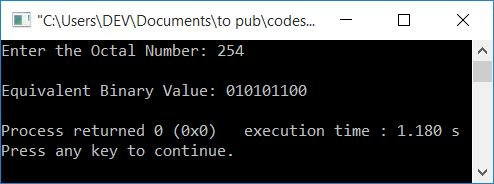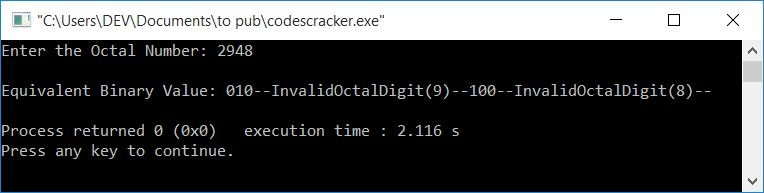# C++ Program to Convert Octal to Binary

In this article, you will learn and get code for octal to binary conversion in C++. The first program is created that actually converts and then prints the binary equivalent of an octal number. The second program simply prints the binary equivalent of each octal digit one by one.

Before going through these programs, if you're not aware of the simple steps and formula used for the conversion, you can refer to the octal to binary formula to get all the things you need.

There are two approaches that can be used to create a program for octal to binary conversion in C++:

In indirect conversion, convert the given octal number into its equivalent decimal value first and then convert the decimal to its equivalent binary value. But here, the program is created using direct conversion only. Because you have the ability to approach indirect conversion with yourself. The program for an indirect approach is already given in a separate article.

## Octal to Binary in C++

To convert an octal number to a binary number in C++ programming, you have to ask the user to enter the octal number and then convert it into its equivalent binary number. And finally, display the binary value on the output as shown in the program given below.

The question is: write a program in C++ that converts octal to binary. The answer to this question is given below:

```#include<iostream>
#include<string.h>
using namespace std;
int main()
{
int octalNum, rev=0, rem, chk=0;
char binaryNum = "";
cout<<"Enter the Octal Number: ";
cin>>octalNum;
while(octalNum!=0)
{
rem = octalNum%10;
if(rem>7)
{
chk++;
break;
}
rev = (rev*10) + rem;
octalNum = octalNum/10;
}
if(chk==0)
{
octalNum = rev;
cout<<"\nEquivalent Binary Value: ";
while(octalNum!=0)
{
rem = octalNum%10;
switch(rem)
{
case 0: strcat(binaryNum, "000");
break;
case 1: strcat(binaryNum, "001");
break;
case 2: strcat(binaryNum, "010");
break;
case 3: strcat(binaryNum, "011");
break;
case 4: strcat(binaryNum, "100");
break;
case 5: strcat(binaryNum, "101");
break;
case 6: strcat(binaryNum, "110");
break;
case 7: strcat(binaryNum, "111");
break;
}
octalNum = octalNum/10;
}
cout<<binaryNum;
}
else
cout<<"\nInvalid Octal Digit!";
cout<<endl;
return 0;
}```

This program was built and runs under the Code::Blocks IDE. Here is its sample run:Now supply any octal number, say 364, as input and then press the ENTER key to convert and print its binary equivalent as shown in the output given below:The function strcat() is used to concatenate strings. It takes two strings as its argument. The value of the second string gets appended at the end of the first string.

The following block of code is included in the above program:

```while(octalNum!=0)
{
rem = octalNum%10;
if(rem>7)
{
chk++;
break;
}
rev = (rev*10) + rem;
octalNum = octalNum/10;
}```

is used to check whether any digit of a given octal number is greater than 7 or not. If any digit is found to be greater than 7, then that digit is not a valid octal digit. Because an octal number can only have eight digits (ranging from 0 to 7),

The while loop executes in the following ways (supposing user input as 364):

• The condition octalNum!=0 or 364!=0 evaluates to be true, therefore program flow goes inside the loop.
• There, octalNum%10 or 364%10 or 4 is set to rem.
• And the condition of if, i.e., rem>7 evaluates to be false, therefore, program flow does not goes inside if's body, but rather to the statement that is available after the if's block of code.
• That is, (rev*10)+rem or (0*10)+4 or 4 gets initialized to rev.
• And the while loop's final statement is evaluated, which means that octalNum/10 or 364/10 or 36 is initialized to octalNum.
• Program flow goes back and evaluates the condition of the while loop again.
• The evaluation of the while loop continues until the value of octalNum becomes equal to 0. That is the condition that evaluates to false.
• Because no any digit in 364 is greater than 7, program flow never goes inside the if's body.
• That means the value of chk is 0 (initialized at the start of the program).
• Therefore, after the ending of the while loop, we've checked whether the value of chk holds its initial value (0) or not.
• If it holds its initial value, then process further to convert the octal number to binary. Otherwise, print a message like "An Invalid Octal Digit was Entered!"
• Now let's perform the dry run of all the blocks of codes inside the if(chk==0) block, given below.

So the dry run of the above program with user input 364 goes like this: (Inside the if block):

• binaryNum="", rev=463 (as I reversed the number earlier using the while loop)
• The value of rev (463) gets initialized to octalNum. So octalNum=463
• Now the condition of the while loop (inside if) gets evaluated.
• That is, the condition octalNum!=0 or 463!=0 evaluates to be true, therefore program flow goes inside the loop, and octalNum%10 or 463%10 or 3 gets initialized to rem.
• The value of rem is now compared with all 8 cases using switch. That is, it has a value of 0, 1, 2, ...., or 7.
• Whatever its value is, concatenates its binary equivalent to the binaryNum variable.
• Because the value of rem is 3, therefore, case 3's statement gets evaluated. That is,
`strcat(binaryNum, "011");`
gets evaluated. Therefore, binaryNum=011 (since the initial value of binaryNum is empty). The binary equivalent will be added after 011 starting next time.
• And octalNum/10, 463/10, or 46 is set to octalNum. So the new value of octalNum is 46.
• Now program flow goes back and evaluates the condition of the while loop again with a new value of octalNum.
• That is, the condition octalNum!=0 or 46!=0 evaluates to be true, and program flow again goes inside the loop.
• The process continues until the condition of the while loop evaluates to be false.
• On continuing the process of evaluating the while loop's block of code, we will get values as follows:
• rem=3, binaryNum=011, octalNum=46
• rem=6, binaryNum=011110, octalNum=4
• rem=4, binaryNum=011110100, octalNum=0
• Now print the value of binaryNum on output; that will be the binary equivalent (011110100) of the given octal number (364).

### In C++, print the binary equivalent of an octal number

Now let's create another program that only prints the binary equivalent of an octal number given by the user at run-time without actually converting it.

```#include<iostream>
using namespace std;
int main()
{
int octalNum, rev=0, rem;
cout<<"Enter the Octal Number: ";
cin>>octalNum;
while(octalNum!=0)
{
rem = octalNum%10;
rev = (rev*10) + rem;
octalNum = octalNum/10;
}
octalNum = rev;
cout<<"\nEquivalent Binary Value: ";
while(octalNum!=0)
{
rem = octalNum%10;
switch(rem)
{
case 0: cout<<"000";
break;
case 1: cout<<"001";
break;
case 2: cout<<"010";
break;
case 3: cout<<"011";
break;
case 4: cout<<"100";
break;
case 5: cout<<"101";
break;
case 6: cout<<"110";
break;
case 7: cout<<"111";
break;
default: cout<<"--InvalidOctalDigit("<<rem<<")--";
break;
}
octalNum = octalNum/10;
}
cout<<endl;
return 0;
}```

Here is its sample run with user input 254 (an octal number):If the user enters an octal number that contains one or more invalid octal digits, say 2948, Then here is the output you'll see:#### The same program in different languages

C++ Quiz

« Previous Program Next Program »